Updated by pat6oct1988 on Jun 01, 2018
REPORT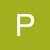pat6oct1988
Owner
6 items   1 followers   0 votes   2 views

Six interesting puzzles to solve in your leisure

Here are some cool interesting puzzles to solve. This puzzles will make your time enjoyable.

Source: http://twominfun.com

1

find six differences in the two imagesThis is one of the popular picture puzzle. In this puzzle, two similar pictures are given with some differences. You need to find those spots where they are different. This type of puzzles can be easily found in newspapers and magazines

2

Math Puzzle: Solve the equations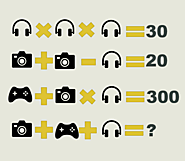In this puzzle several equation on images are given. You need to find the value of the unsolved equation. This is one of most popular puzzle of recent time. They have got lot of attention in news also.

3

Find following words in the word grid: IM, VOIP, GTALK, CHAT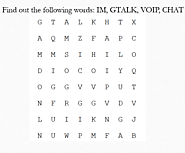Word Search puuzles are also very popular due to its simplicity. In this puzzle, a grid of letters are given you need to find the given words. This puzzles are easy and super fun to solve

4

Find the missing number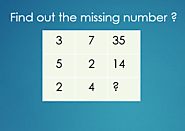Missing number puzzle are very popular logical puzzle. This puzzles are very common in different competitive examinations also. In this puzzle you need to understand the logic behind the given numbers so that you can guess the missing number.

5

Guess the word from images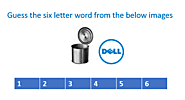This is another interesting picture-word puzzle. Here two images are given, you need to guess the word from these two images.

6

Complete the English Idiom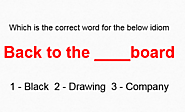Test your English with this puzzle. A Popular english idiom is given. You need to complete it with the appropriate word given in the options.